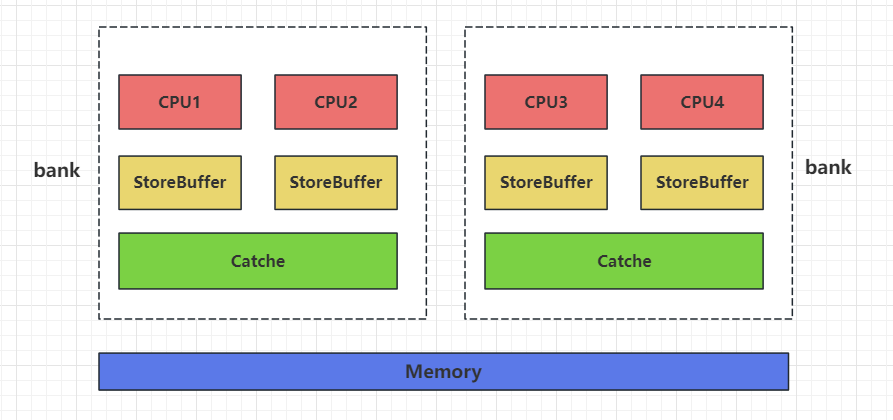# 恋恋风辰的官方博客

## memory_order_seq_cst

`memory_order_seq_cst`代表全局一致性顺序，可以用于 `store`, `load``read-modify-write` 操作, 实现 `sequencial consistent` 的顺序模型. 在这个模型下, 所有线程看到的所有操作都有一个一致的顺序, 即使这些操作可能针对不同的变量, 运行在不同的线程.

``std::atomic<bool> x, y;``std::atomic<int> z;``void write_x_then_y() {``    x.store(true, std::memory_order_relaxed);  // 1``    y.store(true, std::memory_order_relaxed);  // 2``}``void read_y_then_x() {``    while (!y.load(std::memory_order_relaxed)) { // 3``        std::cout << "y load false" << std::endl;``    }``    if (x.load(std::memory_order_relaxed)) { //4``        ++z;``    }``}``void TestOrderRelaxed() {``    std::thread t1(write_x_then_y);``    std::thread t2(read_y_then_x);``    t1.join();``    t2.join();``    assert(z.load() != 0); // 5``}``

``void write_x_then_y() {``    x.store(true, std::memory_order_seq_cst);  // 1``    y.store(true, std::memory_order_seq_cst);  // 2``}``void read_y_then_x() {``    while (!y.load(std::memory_order_seq_cst)) { // 3``        std::cout << "y load false" << std::endl;``    }``    if (x.load(std::memory_order_seq_cst)) { //4``        ++z;``    }``}``void TestOrderSeqCst() {``    std::thread t1(write_x_then_y);``    std::thread t2(read_y_then_x);``    t1.join();``    t2.join();``    assert(z.load() != 0); // 5``}``

## memory_order_relaxed

``void TestOrderRelaxed() {``    std::atomic<bool> rx, ry;``    std::thread t1([&]() {``        rx.store(true, std::memory_order_relaxed); // 1``        ry.store(true, std::memory_order_relaxed); // 2``        });``    std::thread t2([&]() {``        while (!ry.load(std::memory_order_relaxed)); //3``        assert(rx.load(std::memory_order_relaxed)); //4``        });``    t1.join();``    t2.join();``}``

t2线程中3先于4（sequence before），那么1 操作先行于 4. 也就是1 操作的结果可以立即被4获取。进而不会触发断言。

## Acquire-Release

1. 如果使用 memory_order_acquire, 则作为 acquire 操作;
2. 如果使用 memory_order_release, 则作为 release 操作;
3. 如果使用 memory_order_acq_rel, 则同时为两者.

Acquire-release 可以实现 synchronizes-with 的关系. 如果一个 acquire 操作在同一个原子变量上读取到了一个 release 操作写入的值, 则这个 release 操作 “synchronizes-with” 这个 acquire 操作.

``void TestReleaseAcquire() {``    std::atomic<bool> rx, ry;``    std::thread t1([&]() {``        rx.store(true, std::memory_order_relaxed); // 1``        ry.store(true, std::memory_order_release); // 2``        });``    std::thread t2([&]() {``        while (!ry.load(std::memory_order_acquire)); //3``        assert(rx.load(std::memory_order_relaxed)); //4``        });``    t1.join();``    t2.join();``}``

t1执行到2将ry 设置为true, 因为使用了Acquire-release 顺序， 所以 t2 执行到3时读取ry为true， 因此2和3 可以构成同步关系。Acquire-release 的开销比 sequencial consistent 小. 在 x86 架构下, memory_order_acquire 和 memory_order_release 的操作不会产生任何其他的指令, 只会影响编译器的优化: 任何指令都不能重排到 acquire 操作的前面, 且不能重排到 release 操作的后面; 否则会违反 acquire-release 的语义. 因此很多需要实现 synchronizes-with 关系的场景都会使用 acquire-release.

## Release sequences

``void ReleasAcquireDanger2() {``    std::atomic<int> xd{0}, yd{ 0 };``    std::atomic<int> zd;``    std::thread t1([&]() {``        xd.store(1, std::memory_order_release);  // (1)``        yd.store(1, std::memory_order_release); //  (2)``        });``    std::thread t2([&]() {``        yd.store(2, std::memory_order_release);  // (3)``        });``    std::thread t3([&]() {``        while (!yd.load(std::memory_order_acquire)); //（4）``        assert(xd.load(std::memory_order_acquire) == 1); // (5)``        });``    t1.join();``    t2.join();``    t3.join();``}``

1. 同一线程上的写操作
这两种构成的, 则称这一连串的操作为以 release 操作 A 为首的 release sequence. 这里的写操作和 read-modify-write 操作可以使用任意内存顺序.

``void ReleaseSequence() {``    std::vector<int> data;``    std::atomic<int> flag{ 0 };``    std::thread t1([&]() {``        data.push_back(42);  //(1)``        flag.store(1, std::memory_order_release); //(2)``        });``    std::thread t2([&]() {``        int expected = 1;``        while (!flag.compare_exchange_strong(expected, 2, std::memory_order_relaxed)) // (3)``            expected = 1;``        });``    std::thread t3([&]() {``        while (flag.load(std::memory_order_acquire) < 2); // (4)``        assert(data.at(0) == 42); // (5)``        });``    t1.join();``    t2.join();``    t3.join();``}``

t1中操作2是release操作，以2为开始，其他线程(t2)的读改写在release操作之后，我们称之为release sequence， t3要读取release sequence写入的值，所以我们称t1的release操作 “synchronizes with “ t3的 acquire 操作。

## memory_order_consume

`memory_order_consume` 其实是 `acquire-release` 模型的一部分, 但是它比较特殊, 它涉及到数据间相互依赖的关系. 就是前文我们提及的 `carries dependency``dependency-ordered before`.

``p++;   // (1)``i++;   // (2)``p[i]   // (3)``

(1) “sequenced-before” (2), (2) “sequenced-before” (3), 而(1)和(2)的值作为(3)的下表运算符[]的操作数。

memory_order_consume 可以用于 load 操作. 使用 memory_order_consume 的 load 称为 consume 操作. 如果一个 consume 操作在同一个原子变量上读到了一个 release 操作写入的值, 或以其为首的 release sequence 写入的值, 则这个 release 操作 “dependency-ordered before” 这个 consume 操作.

``void ConsumeDependency() {``    std::atomic<std::string*> ptr;``    int data;``    std::thread t1([&]() {``        std::string* p = new std::string("Hello World"); // (1)``        data = 42; // (2)``        ptr.store(p, std::memory_order_release); // (3)``        });``    std::thread t2([&]() {``        std::string* p2;``        while (!(p2 = ptr.load(std::memory_order_consume))); // (4)``        assert(*p2 == "Hello World"); // (5)``        assert(data == 42); // (6)``        });``    t1.join();``    t2.join();``}``

t2执行到(4)处时，需要等到ptr非空才能退出循环，这就依赖t1执行完(3)操作。

## 单例模式改良

``//利用智能指针解决释放问题``class SingleAuto``{``private:``    SingleAuto()``    {``    }``    SingleAuto(const SingleAuto&) = delete;``    SingleAuto& operator=(const SingleAuto&) = delete;``public:``    ~SingleAuto()``    {``        std::cout << "single auto delete success " << std::endl;``    }``    static std::shared_ptr<SingleAuto> GetInst()``    {``        // 1 处``        if (single != nullptr)``        {``            return single;``        }``        // 2 处``        s_mutex.lock();``        // 3 处``        if (single != nullptr)``        {``            s_mutex.unlock();``            return single;``        }``        // 4处``        single = std::shared_ptr<SingleAuto>(new SingleAuto);``        s_mutex.unlock();``        return single;``    }``private:``    static std::shared_ptr<SingleAuto> single;``    static std::mutex s_mutex;``};``

``std::shared_ptr<SingleAuto> SingleAuto::single = nullptr;``std::mutex SingleAuto::s_mutex;``void TestSingle() {``    std::thread t1([]() {``        std::cout << "thread t1 singleton address is 0X: " << SingleAuto::GetInst() << std::endl;``        });``    std::thread t2([]() {``        std::cout << "thread t2 singleton address is 0X: " << SingleAuto::GetInst() << std::endl;``        });``    t2.join();``    t1.join();``}``

1处和4处代码存在线程安全问题，因为4处代码在之前的文章中我谈过，new一个对象再赋值给变量时会存在多个指令顺序

``1 为对象allocate一块内存空间``2 调用construct构造对象``3 将构造到的对象地址返回``

``1 为对象allocate一块内存空间``2 先将开辟的空间地址返回``3 调用construct构造对象``

``//利用智能指针解决释放问题``class SingleMemoryModel``{``private:``    SingleMemoryModel()``    {``    }``    SingleMemoryModel(const SingleMemoryModel&) = delete;``    SingleMemoryModel& operator=(const SingleMemoryModel&) = delete;``public:``    ~SingleMemoryModel()``    {``        std::cout << "single auto delete success " << std::endl;``    }``    static std::shared_ptr<SingleMemoryModel> GetInst()``    {``        // 1 处``        if (_b_init.load(std::memory_order_acquire))``        {``            return single;``        }``        // 2 处``        s_mutex.lock();``        // 3 处``        if (_b_init.load(std::memory_order_relaxed))``        {``            s_mutex.unlock();``            return single;``        }``        // 4处``        single = std::shared_ptr<SingleMemoryModel>(new SingleMemoryModel);``        _b_init.store(true, std::memory_order_release);``        s_mutex.unlock();``        return single;``    }``private:``    static std::shared_ptr<SingleMemoryModel> single;``    static std::mutex s_mutex;``    static std::atomic<bool> _b_init ;``};``std::shared_ptr<SingleMemoryModel> SingleMemoryModel::single = nullptr;``std::mutex SingleMemoryModel::s_mutex;``std::atomic<bool> SingleMemoryModel::_b_init = false;``

``void TestSingleMemory() {``    std::thread t1([]() {``        std::cout << "thread t1 singleton address is 0x: " << SingleMemoryModel::GetInst() << std::endl;``        });``    std::thread t2([]() {``        std::cout << "thread t2 singleton address is 0x: " << SingleMemoryModel::GetInst() << std::endl;``        });``    t2.join();``    t1.join();``}``

## 总结

https://gitee.com/secondtonone1/boostasio-learn/tree/master/concurrent/day11-AcquireRelease

https://space.bilibili.com/271469206/channel/collectiondetail?sid=1623290

#### 热门文章

1. Linux环境搭建和编码

喜欢(594) 浏览(3732)
2. windows环境搭建和vscode配置

喜欢(587) 浏览(1496)
3. slice介绍和使用

喜欢(521) 浏览(1448)
4. 解密定时器的实现细节

喜欢(566) 浏览(1340)
5. C++ 类的继承封装和多态

喜欢(588) 浏览(1859)

#### 最新评论

1. Linux环境搭建和编码 恋恋风辰:Linux环境下go的安装比较简单，可以不用设置GOPATH环境变量，后期我们学习go mod 之后就拜托了go文件目录的限制了。
2. 构造函数 secondtonone1:构造函数是类的基础知识，要着重掌握
3. visual studio配置boost库 Mr.test:visual studio时 应该使用  \b2.exe toolset=msvc”  🤡
4. 类和对象 陈宇航:支持！！！！
5. slice介绍和使用 恋恋风辰:切片作为引用类型极大的提高了数据传递的效率和性能，但也要注意切片的浅拷贝隐患，算是一把双刃剑，这世间的常态就是在两极之间寻求一种稳定。

#### 个人公众号#### 个人微信